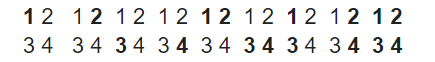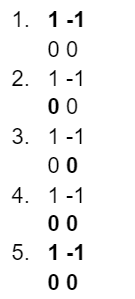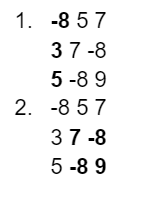5

# Sub Matrices With Sum Zero

Difficulty: MEDIUMContributed By
Dhruv Sharma
Avg. time to solve
15 min
Success Rate
85%

Problem Statement
Suggest Edit

#### NOTE:

``````1. The matrix may also contain some negative elements.
2. A square matrix is a matrix with the same number of rows and columns.
A matrix obtained by deleting some (possibly zero) of the rows and/or columns from the beginning and/or from the end of a matrix is said to be a sub-matrix of the given matrix.

Example: Given a matrix
A = 1 2
3 4

Possible non-empty sub-matrices of A are represented below by bold numbers-
``````##### Input Format:
``````The first line of input contains T, denoting the number of test cases.

The first line of each test case contains an integer N, the order of the square matrix.

The following N lines contain N space-separated integers, representing the elements in the ith row of the matrix 'MAT'.
``````
##### Output Format:
``````The only line of output of each test case should contain an integer denoting the number of non-empty sub-matrices such that the sum of all the elements inside the sub-matrix is equal to zero.
``````
##### Note:
``````You don’t need to print anything. It has already been taken care of. Just implement the given function.
``````
##### Constraints:
``````1 <= T <= 5
1 <= N <= 70

Time Limit: 1 sec
``````
##### Sample Input 1:
``````1
2
1 -1
0 0
``````
##### Sample Output 1:
``````5
``````
##### Explanation of Sample Input 1:
``````As there are only 5 submatrices whose sum is equal to 0, we get our answer to be 0. The submatrices are as follows, the elements in bold represent the elements that are in that submatrix.
``````##### Sample Input 2:
``````1
3
-8 5  7
3  7 -8
5 -8  9
``````
##### Sample Output 2:
``````2
``````
##### Explanation of Sample Input 2:
``````As there are only 2 submatrices whose sum is equal to 0, we get our answer to be 0. The submatrices are as follows, the elements in bold represent the elements that are in that submatrix.
``````Console